# Vibration of a simply supported graphene sheet with uncertain small scale parameter based on nonlocal theory

## G. Q. Xie1, S. S. Ni2

1, 2Civil Engineering College, Hunan University of Science and Technology, Xiangtan, 411201, P. R. China

1Corresponding author

Mathematical Models in Engineering, Vol. 7, Issue 2, 2021, p. 22-29. https://doi.org/10.21595/mme.2021.21982
Received 8 April 2021; received in revised form 16 April 2021; accepted 28 April 2021; published 30 June 2021

Copyright © 2021 G. Q. Xie, et al. This is an open access article distributed under the Creative Commons Attribution License, which permits unrestricted use, distribution, and reproduction in any medium, provided the original work is properly cited.
Editor's Pick
Views 57
CrossRef Citations 0
Abstract.

Small scale parameter of graphene sheet is considered as uncertain one, vibration equation of a simply supported graphene sheet with uncertainty is established based on nonlocal theory. Trigonometric function series solution and interval operator are used to obtain the upper and lower bound of response of the simply supported graphene sheet. the uncertainty level of response for the different dimension is investigated. The numerical result shows that for the same uncertainty level of small scale parameter, the uncertainty level of the response will decrease with increase of the graphene sheet dimension, and a small uncertainty level of the small scale parameter can cause much greater uncertainty level of the response before the small scale effect disappears.

Highlights
• Comparison of the above maximum uncertainty level of the different dimension graphene sheet can be concluded that for the same uncertainty level of small scale parameter, the uncertainty level of the response will decrease with increase of the graphene sheet dimension.
• It is found that a small uncertainty level of the small scale parameter can cause much greater uncertainty level of the response before the small scale effect disappears.
• We found from the previous study  that the small scale effect will disappear if the side lengths of the graphene sheet are both larger than 1um, the result is agreement with that given in this paper.

Keywords: graphene sheet, uncertain parameter, interval variable, nonlocal theory.

#### 1. Introduction

With the development of nano mechanical and electrical technology, the mechanical properties of nanoscale structures to cause the considerable attention of many scholars. The small scale effect is found during study of nano structures. Due to the surface effect and the small-scale effect of nanomaterials, classical continuum mechanics will lead to an inaccurate result when it is used to solve the mechanics problem of nanomaterials. Fortunately, the nonlocal theory given by Eringen  can remove the shortcoming of classical continuum mechanics. Based on the nonlocal theory, Zhang, Liu, and Wang  studied the buckling of multi-walled carbon nanotube. Xie, Han, and Long [3-5] investigated the small scale effect and the vibration of carbon nanotube. Wang  used a modified nonlocal beam model to study vibration and stability of nanotubes conveying fluid. Hybrid nonlocal beam model  was employed to study bending, buckling, and vibration of micro/nanobeams. Liang Y. and Han Q.  gave prediction of the nonlocal scaling parameter for graphene sheet. L Yang，J S Peng  used the nonlocal-gradient elasticity theory to scale effect on dynamic analysis of electrostatically actuated nano beams. Hamid M. et al.  studied the size dependent static and dynamic pull-in instability of cantilever nanoactuator based on strain gradient theory. Fang B., Zhen Y. X., Zhang C. P., et al.  carried out nonlinear vibration analysis of double-walled carbon nanotubes based on nonlocal elasticity theory. Reddy J. N.  presented nonlocal nonlinear formulations for bending of classical and shear deformation theories of beams and plates. M. Ghalambaz  used energy balance method to investigate nonlinear oscillation of nanoelectro-mechanical resonators.

In previous studies, the parameters of the graphene sheet are deterministic. However, until now, the deterministic small scale parameter of the graphene sheet has been given. This will lead to the uncertain dynamic response of the graphene sheet.

In this paper, a nonlocal model of nanoplate is developed for vibration of a simply supported graphene sheet with uncertainty. The upper and lower bound of response of a clamped graphene sheet is obtained, and the uncertainty level of response is also obtained.

#### 2.1. Nonlocal constitutive equation

The nonlocal constitutive equations of graphene  are:

(1)
$\left\{\begin{array}{l}{\sigma }_{11}-{\left({e}_{0}a\right)}^{2}\frac{{\partial }^{2}{\sigma }_{11}}{\partial {x}_{1}^{2}}=\frac{E}{1-{\mu }^{2}}\left[{\epsilon }_{11}+\mu {\epsilon }_{22}\right],\\ {\sigma }_{22}-{\left({e}_{0}a\right)}^{2}\frac{{\partial }^{2}{\sigma }_{22}}{\partial {x}_{2}^{2}}=\frac{E}{1-{\mu }^{2}}\left[{\epsilon }_{22}+\mu {\epsilon }_{11}\right],\\ \left(1-{\left({e}_{0}a\right)}^{2}{\nabla }^{2}\right){\sigma }_{12}=\frac{E}{1+\mu }{\epsilon }_{12},\end{array}\right\$

where $E$ is the elastic modulus of graphene, and $\mu$ Poisson’s ratio, $a$ an internal characteristic length (C-C bond length), ${e}_{0}$ a constant appropriate to each material considered as the uncertain parameter.

Eq. (1) can be approximately expressed as:

(2)
$\left\{\begin{array}{l}{\sigma }_{11}=\frac{E}{1-{\mu }^{2}}\left[1+{\left({e}_{0}a\right)}^{2}\frac{{\partial }^{2}}{\partial {x}_{1}^{2}}\right]\left[{\epsilon }_{11}+\mu {\epsilon }_{22}\right],\\ {\sigma }_{22}=\frac{E}{1-{\mu }^{2}}\left[1+{\left({e}_{0}a\right)}^{2}\frac{{\partial }^{2}}{\partial {x}_{3}^{2}}\right]\left[{\epsilon }_{22}+\mu {\epsilon }_{11}\right],\\ {\sigma }_{12}=\frac{E}{1+\mu }\left[1+{\left({e}_{0}a\right)}^{2}{\nabla }^{2}\right]{\epsilon }_{12}.\end{array}\right\$

#### 2.2. Geometric equations

Eringen  proposed the nonlocal theory in which the nonlocality of stress is mainly considered and strain is remained the same as the classical. three displacement components can be expressed in terms of the deflection based on the Kirchhoff assumption. The relationship between the three components of strain and deflection can be given by:

(3)
$\begin{array}{l}{\epsilon }_{11}=-z\frac{{\partial }^{2}w}{\partial {{x}_{1}}^{2}},\\ {\epsilon }_{22}=-z\frac{{\partial }^{2}w}{\partial {{x}_{2}}^{2}},\\ {\epsilon }_{12}=-2z\frac{{\partial }^{2}w}{\partial {x}_{1}\partial {x}_{2}}.\end{array}$

#### 2.3. The governing equation of motion

Hamilton principle is used to establish the governing equation of motion, mathematically, Hamilton principle states:

(4)
$\delta {\int }_{{t}_{1}}^{{t}_{2}}L\mathrm{d}t=0,$

where $L$ is Lagrangian function. $L$ is given as:

(5)
$L=T-U+W,$

where $T$ is the kinetic energy, $U$ the strain energy, and $W$ the work done by the external forces.

The kinetic energy is given by:

(6)
$T=\frac{1}{2}{\iint }_{A}\stackrel{-}{m}{\stackrel{˙}{w}}^{2}dxdy,$

where $\stackrel{-}{m}$ is the mass density of unit area, $A$ stands for the whole area of the graphene sheet.

The strain energy of the graphene sheet can be expressed as:

(7)
$U=\frac{1}{2}{\iiint }_{V}\sigma {\epsilon }^{T}dV.$

The work done by the external force can be obtained by:

(8)
$W={\iint }_{A}\left(f-c\stackrel{˙}{w}\right)wdxdy,$

where $f$ is the distribution force on the upper surface of the graphene sheet, $c$ stands for damping coefficient.

Substitution of Eqs. (5)-(8) into Eq. (4) yields the governing equation of motion:

(9)
${\left({e}_{0}a\right)}^{2}\left(\frac{{\partial }^{6}w}{\partial {{x}_{1}}^{6}}+\frac{{\partial }^{6}w}{\partial {{x}_{2}}^{6}}\right)+{\left({e}_{0}a\right)}^{2}\left(\frac{{\partial }^{6}w}{\partial {{x}_{1}}^{4}\partial {{x}_{2}}^{2}}+\frac{{\partial }^{6}w}{\partial {{x}_{1}}^{2}\partial {x}_{2}^{4}}\right)+{\nabla }^{4}w+\frac{\stackrel{-}{m}}{D}\stackrel{¨}{w}+\frac{c}{D}\stackrel{˙}{w}=\frac{f}{D},$

where $D$ is the flexural rigidity $D=\frac{E{h}^{3}}{12\left(1-{\mu }^{2}\right)}$, $h$ thickness of the graphene sheet.

#### 2.4. Solution of the governing equation of motion

Transient response of Eq. (9) satisfying the simply supported boundary can be given by:

(10)
${w}_{1}={e}^{st}\mathrm{s}\mathrm{i}\mathrm{n}\frac{m\pi x}{{l}_{x}}\mathrm{s}\mathrm{i}\mathrm{n}\frac{n\pi y}{{l}_{y}},$

where ${l}_{x}$ and ${l}_{y}$ denote the length and width of the graphene sheet, respectively.

Substitution of Eq. (10) into Eq. (9) yields the transient response:

(11)

where damping frequency ${\omega }_{d}^{mn}$:

(12)
${\omega }_{d}^{mn}=\sqrt{1-{\zeta }^{2}}{\omega }_{mn}.$

The undamped natural frequency ${\omega }_{mn}$:

(13)
${\omega }_{mn}=\sqrt{\frac{D}{\stackrel{-}{m}}\left[{\left({e}_{0}a\right)}^{2}\left(\frac{{m}^{6}{\pi }^{6}}{{l}_{x}^{6}}+\frac{{n}^{6}{\pi }^{6}}{{l}_{y}^{6}}+\frac{{m}^{4}{n}^{2}{\pi }^{6}}{{l}_{x}^{4}{l}_{y}^{2}}+\frac{{m}^{2}{n}^{4}{\pi }^{6}}{{l}_{x}^{2}{l}_{y}^{4}}\right)+{\left(\frac{{m}^{2}{\pi }^{2}}{{l}_{x}^{2}}+\frac{{n}^{2}{\pi }^{2}}{{l}_{y}^{2}}\right)}^{2}\right]}.$

Damping ratio $\zeta$:

(14)
$\zeta =\frac{c}{2\stackrel{-}{m}{\omega }_{mn}}.$

The initial condition:

(15)
$w={w}_{0},\begin{array}{ll}& \stackrel{˙}{w}={\stackrel{˙}{w}}_{0}\end{array}.$

Combination of Eq. (15) with Eq. (11), has:

(16)
(17)

Transient response:

(18)
${w}_{1}={\sum }_{m=1,3,5\cdots }^{\infty }{\sum }_{n=1,3,5\cdots }^{\infty }\frac{16{e}^{-\zeta {\omega }_{mn}t}}{mn{\pi }^{2}}\left[\frac{{\stackrel{˙}{w}}_{0}+\zeta {\omega }_{mn}{w}_{0}}{{\omega }_{d}^{mn}}\mathrm{s}\mathrm{i}\mathrm{n}\left({\omega }_{d}^{mn}t\right)+{\stackrel{˙}{w}}_{0}\mathrm{c}\mathrm{o}\mathrm{s}\left({\omega }_{d}^{mn}t\right)\right]\mathrm{s}\mathrm{i}\mathrm{n}\frac{m\pi x}{{l}_{x}}\mathrm{s}\mathrm{i}\mathrm{n}\frac{n\pi y}{{l}_{y}}.$

Let a harmonic excitation $f={q}_{0}\mathrm{s}\mathrm{i}\mathrm{n}\omega t$ is applied on the graphene sheet, the steady response of the graphene sheet can be adopted as the following form:

(19)
${w}_{2}={\sum }_{m=1}^{\infty }{\sum }_{n=1}^{\infty }W\left(t\right)\mathrm{s}\mathrm{i}\mathrm{n}\frac{m\pi x}{{l}_{x}}\mathrm{s}\mathrm{i}\mathrm{n}\frac{n\pi y}{{l}_{y}}.$

Substituting Eq. (19) and $f$ into Eq. (9) and then solving Eq. (9) based on orthogonal property of trigonometric function yields:

(20)

where:

(21)
$\phi =\mathrm{t}\mathrm{a}{\mathrm{n}}^{-1}\frac{c\omega }{k-\stackrel{-}{m}{\omega }^{2}},$
(22)
$k=D\left[{\left({e}_{0}a\right)}^{2}\left(\frac{{m}^{6}{\pi }^{6}}{{l}_{x}^{6}}+\frac{{n}^{6}{\pi }^{6}}{{l}_{y}^{6}}+\frac{{m}^{4}{n}^{2}{\pi }^{6}}{{l}_{x}^{4}{l}_{y}^{2}}+\frac{{m}^{2}{n}^{4}{\pi }^{6}}{{l}_{x}^{2}{l}_{y}^{4}}\right)+{\left(\frac{{m}^{2}{\pi }^{2}}{{l}_{x}^{2}}+\frac{{n}^{2}{\pi }^{2}}{{l}_{y}^{2}}\right)}^{2}\right].$

The total response is:

(23)
$w={w}_{1}+{w}_{2}.$

#### 2.5. Interval analysis of vibration response

Let ${v}^{I}$ is an interval variable, the upper and lower bound of ${v}^{I}$ can be expressed as:

(24)

where ${v}^{m}$ is the midpoint value of ${v}^{I}$, ${v}^{r}$ the interval radius of ${v}^{I}$.

The interval variable ${v}^{I}$ is between the upper and lower bound:

(25)
${v}^{I}\in \left[\stackrel{-}{v},\underset{_}{v}\right].$

$w$ can be expanded into Taylor series:

(26)
$w={w}_{1}\left({v}^{m}\right)+\frac{\partial {w}_{1}\left({v}^{m}\right)}{{v}^{I}}\left({v}^{I}-{v}^{m}\right)+\cdots +{w}_{2}\left({v}^{m}\right)+\frac{\partial {w}_{2}\left({v}^{m}\right)}{{v}^{I}}\left({v}^{I}-{v}^{m}\right)+\cdots .$

The midpoint value of response $w$:

(27)

The interval radius of response $w$:

(28)

#### 3. Numerical example

For all the subsequent numerical example, the length of C-C bond $a=$ 0.142×10-9 m, in-plane stiffness $Eh=$ 360 J/m2 (Sanchez -Portal,D, ), Poisson’s ratio $\mu =$ 0.26, the thickness of the graphene sheet $h=$ 0.34×10-9 m, the mass density of unit area $\stackrel{-}{m}=$ 0.77×10-3 kg/m2, the damping coefficient 0.3 Ns/m. The initial velocity ${\stackrel{˙}{w}}_{0}=0$, the initial deflection ${w}_{0}=$ 5×10-9 m.

Let ${e}_{0}$ is an interval variable, the uncertainty level ±3 % off from the midpoints 0.39, investigated. The amplitude of load is 300 nN/m2, the uncertainty level of response for the different dimension graphene sheet is investigated.

Dynamic response bounds of deflection of the center point of the sheet ${l}_{x}=$ 2×10-2 m, ${l}_{y}=$ 1×10-2 m) is shown in Fig. 1(a). From this figure, it can be found that the amplitude of vibration of the sheet obeys law of exponent decay. The maximum uncertainty level of response off from the midpoints is 2.393 %. The maximum uncertainty level of response is smaller than the uncertainty level ±3 % of ${e}_{0}$.

Dynamic response bounds of deflection of the center point of the sheet (${l}_{x}=$ 2×10-3 m, ${l}_{y}=$ 1×10-3) mis shown in Fig. 1(b). From this figure, it can be found that the amplitude of vibration of the sheet obeys law of exponent decay. The maximum uncertainty level of response off from the midpoints is 10.733 %. The maximum uncertainty level of response is 3 times more than the uncertainty level ±3 % of ${e}_{0}$.

Dynamic response bounds of deflection of the center point of the sheet (${l}_{x}=$ 2×10-4 m, ${l}_{y}=$ 1×10-4 is shown in Fig. 1(c). From this figure, it can be found that the amplitude of vibration of the sheet obeys law of exponent decay. The maximum uncertainty level of response off from the midpoints arrives to 45.423 %. The maximum uncertainty level of response is 15 times more than the uncertainty level ±3 % of ${e}_{0}$.

Fig. 1. Response bounds of deflection of the center point of the sheet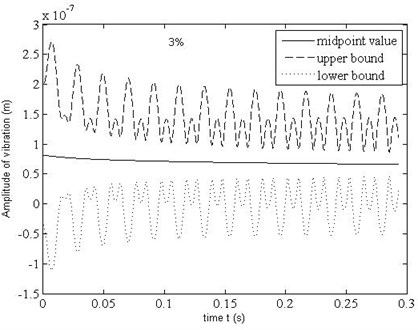a) 2×10-2 m, ${l}_{y}=$ 1×10-2 m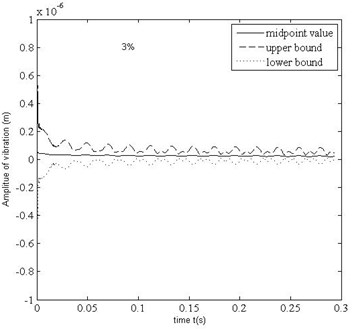b)${l}_{x}=$ 2×10-3 m, ${l}_{y}=$ 1×10-3 m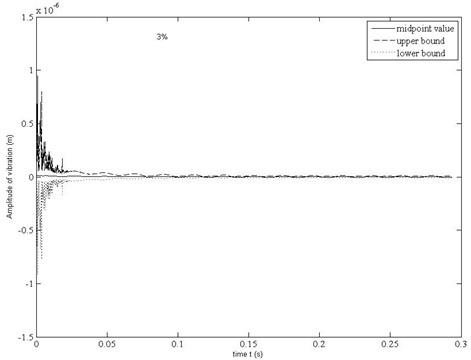c)${l}_{x}=$ 2×10-4 m, ${l}_{y}=$ 1×10-4 m

Fig. 2. Response bounds of deflection of the center point of the sheet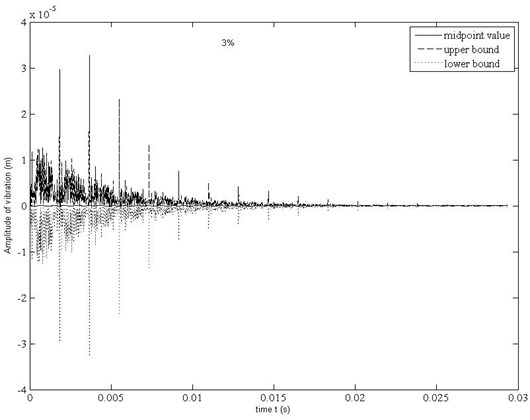a)${l}_{x}=$ 2×10-5 m, ${l}_{y}=$ 1×10-5 m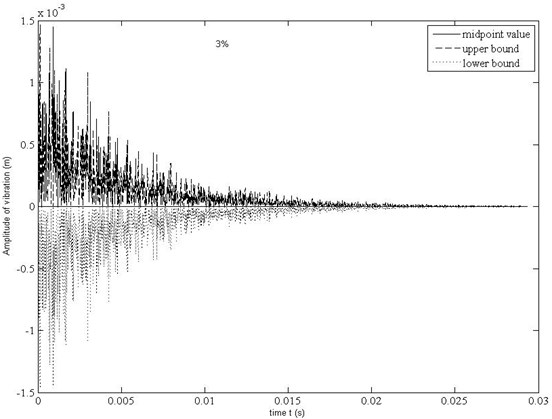b)${l}_{x}=$ 2×10-7 m, ${l}_{y}=$ 1×10-7 m

Dynamic response bounds of deflection of the center point of the sheet (${l}_{x}=$ 2×10-5 m, ${l}_{y}=$ 1×10-5 m) is shown in Fig. 2(a). It can be seen that amplitude of the sheet overall presents the attenuation trend. The maximum uncertainty level of response off from the midpoints arrives to 7.618×105 %.

Dynamic response bounds of deflection of the center point of the sheet (${l}_{x}=$ 2×10-7 m, ${l}_{y}=$ 1×10-7 m) is shown in Fig. 2(b). From this figure, it can also be found that amplitude of the sheet overall presents the attenuation trend. The numerical result shows that the maximum uncertainty level of response off from the midpoints arrives to 3.814×107 %.

#### 4. Conclusions

Comparison of the above maximum uncertainty level of the different dimension graphene sheet can be concluded that for the same uncertainty level of small scale parameter, the uncertainty level of the response will decrease with increase of the graphene sheet dimension. We found from the previous study G. Q. Xie  that the small scale effect will disappear if the side lengths of the graphene sheet are both larger than 1×10-7 m. Here we found that a small uncertainty level of the small scale parameter can cause much greater uncertainty level of the response before the small scale effect disappears.

The result that the small scale effect will disappear if the side lengths of the graphene sheet are both larger than 1×10-7 mis valuable and very helpful for the design and manufacture of graphene and CNT devices are of guiding significance.

#### Acknowledgements

This work is supported by National Natural Science Foundation of China under the Grant Number 11372109.

1. Eringen A. C., Edelen Gb D. On nonlocal elasticity. International Journal of Engineering, Vol. 10, 1972, p. 233-248. [Publisher]
2. Zhang Y. Q., Liu G. R., Wang J. S. Small scale effects on buckling of multi-walled carbon nanotubes under axial compression. Physical Review B, Vol. 70, 2004, p. 205430. [Publisher]
3. Xie G. Q., Han X., Liu G. R., Long S. Y. Effect of small size scale on the radial buckling pressure of a clamped multi-walled carbon nanotube. Smart Materials and Structures, Vol. 15, 2006, p. 1143-1149. [Publisher]
4. Xie G. Q., Long S. Y. Elastic vibration behaviors of carbon nanotubes based on micropolar mechanics. Computers, Materials and Continua, Vol. 4, Issue 2, 2006, p. 11-20. [Search CrossRef]
5. Xie G. Q., Han X., Long S. Y. The effect of the small size on dispersion characteristics of the carbon nanotube. International Journal of Solid and Structure, Vol. 44, 2007, p. 1242-1255. [Publisher]
6. Wang L. A modified nonlocal beam model for vibration and stability of nanotubes conveying fluid. Physica E: Low-dimensional Systems and Nanostructures, Vol. 44, Issue 1, 2011, p. 25-28. [Publisher]
7. Zhang Y. Y., Wang C. M., Challamel N. Bending, buckling, and vibration of micro/nano beams by hybrid nonlocal beam model. Journal of Engineering Mechanics, Vol. 136, Issue 5, 2009, p. 562-574. [Publisher]
8. Liang Y., Han Q. Prediction of the nonlocal scaling parameter for grapheme sheet. European Journal of Mechanics – A/Solids, Vol. 45, 2014, p. 153-160. [Publisher]
9. Peng J. S., Yang L., Yang J. Size effect on the dynamic analysis of electro statically actuated micro-actuators. Microsyst Technol, Vol. 23, 2017, p. 1247-1254. [Publisher]
10. Hamid Sedighi M., Koochi A., Abadyan M. Modeling the size dependent static and dynamic pull-in instability of cantilever nanoactuator based on strain gradient theory. International Journal of Applied Mechanics, Vol. 6, Issue 5, 2014, p. 1450055 [Publisher]
11. Fang B., Zhen Y. X., Zhang C. P., et al. Nonlinear vibration analysis of double-walled carbon nanotubes based on nonlocal elasticity theory. Applied Mathematical Modeling, Vol. 37, Issue 3, 2013, p. 1096-1107. [Publisher]
12. Reddy J. N. Nonlocal nonlinear formulations for bending of classical and shear deformation theories of beams and plates. International Journal of Engineering Science, Vol. 48, Issue 11, 2010, p. 1507-1518. [Publisher]
13. Ghalambaz M., Ghalambaz M., Edalatifar M. Nonlinear oscillation of nanoelectro- mechanical resonators using energy balance method: considering the size effect and the van der Waals force. Applied Nanoscience, Vol. 6, 2016, p. 309-317. [Publisher]
14. Sanchez Portal D., et al. A initio structural, elastic, and vibrational properties of carbon nanotubes, Physical Review B, Vol. 59, 1999, p. 12678-12688. [Publisher]
15. Xie Genquan, Wang Jianping, Zhang Qilong Small scale effect of a simply supported graphene sheet’s dynamic response. Journal of Vibration and Shock, Vol. 37, Issue 15, 2018, p. 277-282. [Search CrossRef]